Open in App
Not now

# How to Convert Categorical Variable to Numeric in Pandas?

• Last Updated : 01 Dec, 2021

In this article, we will learn how to convert a categorical variable into a Numeric by using pandas.

When we look at the categorical data, the first question that arises to anyone is how to handle those data, because machine learning is always good at dealing with numeric values. We could make machine learning models by using text data. So, to make predictive models we have to convert categorical data into numeric form.

## Method 1: Using replace() method

Replacing is one of the methods to convert categorical terms into numeric. For example, We will take a dataset of people’s salaries based on their level of education. This is an ordinal type of categorical variable. We will convert their education levels into numeric terms.

Syntax:

replace(to_replace=None, value=None, inplace=False, limit=None, regex=False, method=’pad’)

Consider the given data: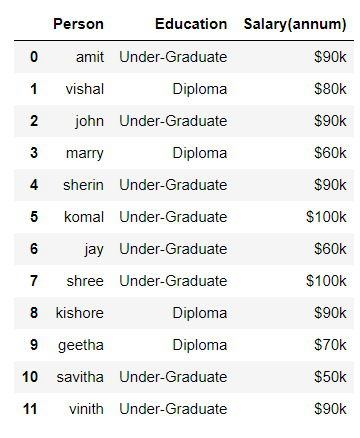Data

## Python3

 `#import pandas``import` `pandas as pd` `# read csv file``df ``=` `pd.read_csv(``'data.csv'``)` `# replacing values``df[``'Education'``].replace([``'Under-Graduate'``, ``'Diploma '``],``                        ``[``0``, ``1``], inplace``=``True``)`

Output: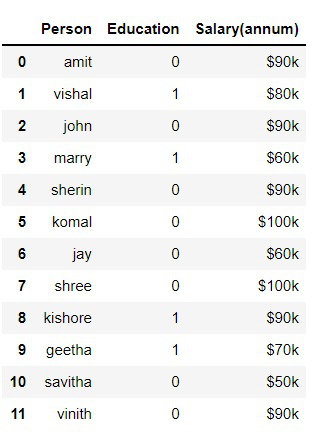In the above program, we have replaced “under-graduate” as 0 and “Diploma” as 1.

## Method 2: Using get_dummies()

Replacing the values is not the most efficient way to convert them. Pandas provide a method called get_dummies which will return the dummy variable columns.

Syntax: pandas.get_dummies(data, prefix=None, prefix_sep=’_’, dummy_na=False, columns=None, sparse=False, drop_first=False, dtype=None)

### Stepwise Implementation

Step 1: Create dummies columns

get_dummies() method is called and the parameter name of the column is given. This method will return the dummy variable columns. In this case, we have 3 types of Categorical variables so, it returned three columns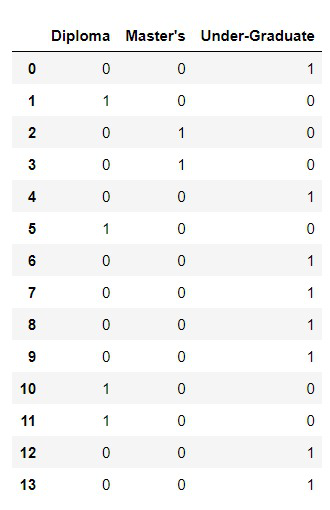Step 2: Concatenate

Syntax: pandas.concat(objs, axis=0, join=’outer’, ignore_index=False, keys=None, levels=None, names=None, verify_integrity=False, sort=False, copy=True

The next step is to concatenate the dummies columns into the data frame. In pandas, there is a concat()  method, which you can call to join two data frames. You should supply it with the name of two data frames and the axis. This will give you the merged data frame.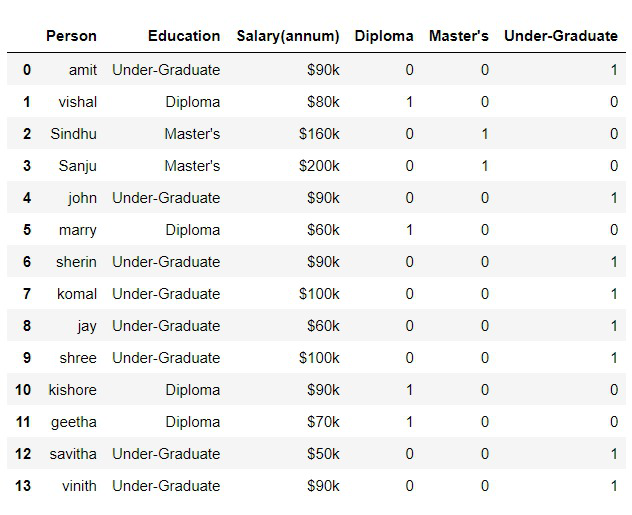Step 3: Drop columns

We have to drop the original ‘education’ column because we have the dummy variable column and we don’t need the text column. And we might also drop one of the dummy variable columns So that we could avoid the dummy variable trap which could mess up the model. After dropping the columns,  the desired dataframe is obtained

We will implement this at code

## Python3

 `#import pandas``import` `pandas as pd` `# read csv``df ``=` `pd.read_csv(``'salary.csv'``)` `# get the dummies and store it in a variable``dummies ``=` `pd.get_dummies(df.Education)` `# Concatenate the dummies to original dataframe``merged ``=` `pd.concat([df, dummies], axis``=``'columns'``)` `# drop the values``merged.drop([``'Education'``, ``'Under-Graduate'``], axis``=``'columns'``)` `# print the dataframe``print``(merged)`

Output: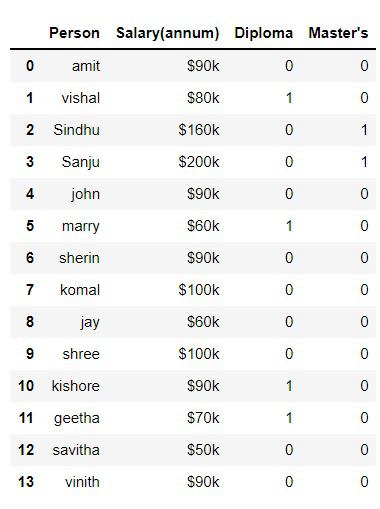My Personal Notes arrow_drop_up